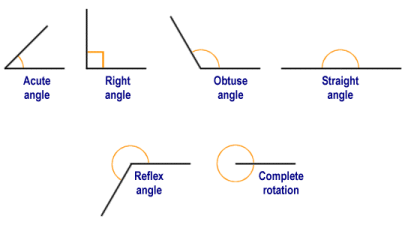# Angle

9th October 2017 Off

The space between two intersecting lines or surfaces at or close to the point where they meet.
It is usually measured in degree.Angle is  measured as the amount of turn.

Type of Angles
There are six different types of angles.

1. Acute angle  is less than 90°.
2. Obtuse angle is greater than 90°.
3. Right angle is exactly  90°.
4. Straight angle is equals to 180°.
5. Reflex angle is greater than 180°.
6. Full Rotation is exactly 360°.Negative and Positive Angles.When measuring from a line:

1. positive angle goes counterclockwise (opposite direction that clocks go).
2. negative angle goes clockwise.

Angles are drawn by the